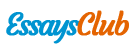# Algebra

• ### Assignment on Equations and Inequations

Note: Solve the assignment and send ONLY the exercises indicated by the teacher 1. Change the following propositions into one variable equations 1. Seven times a number equals five more than the number 2. Seventeen less four times a number equals twenty added to six times the number. 3.

Rating:
Words: 1,966  •  Pages: 8
• ### Independent and Dependent Variable

Independent and dependent variable ========== ﻿Name: Gabriel Garcia de Carvalho 09/07/2014 PH 383 Biostatistics Assignment 1 Due Date: September 9, 2014 1. Provide an explanation/definition of [6 pts] a. Independent Variable: The independent variable is the variable that is able to lead to changes in the status of the dependent

Rating:
Words: 712  •  Pages: 3
• ### Matemathics III

Pia de matematicas ========== ﻿Universidad Autónoma de Nuevo León Resultado de imagen para universidad autonoma de nuevo leon Resultado de imagen para preparatoria 1 PIA MATEMATHICS III Name: José Luis Aguirre Galarza Teacher: Sergio Bustamante Roll Number: 1 ID: 1864016 Group: 301 Introduction In this semester we learned different things

Rating:
Words: 1,493  •  Pages: 6
• ### Ols to Estimate a Model Relating Median Housing Price in the Community to Various Community Characteristics

Question 1 1. ‘Use OLS to estimate a model relating median housing price in the community to various community characteristics given by the equation below and present the estimated equation, including the standard errors of estimated coefficients and R2.’ In order to attain the estimated coefficients, their standard errors and

Rating:
Words: 2,852  •  Pages: 12
• ### Vertical Throw Upwards (conceptual)

Aplication activity ========== ﻿Application Activity Vertical throw upwards (conceptual) In this activity we will work in a conceptual way mathematical expressions) (that means, without the concept of vertical motion. 1. After seeing the topic and do exercises about vertical throw upwards helped by your teacher, you can do this activity.

Rating:
Words: 510  •  Pages: 3